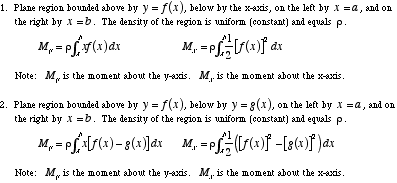index: click on a letter A B C D E F G H I J K L M N O P Q R S T U V W X Y Z A to Z index index: subject areas numbers & symbols sets, logic, proofs geometry algebra trigonometry advanced algebra & pre-calculus calculus advanced topics probability & statistics real world applications multimedia entrieswww.mathwords.com about mathwords website feedback

 Moment A number indicating the degree to which a figure tends to balance on a given line (axis). A moment of zero indicates perfect balance, and a large moment indicates a strong tendency to tip over. Formally, the moment of a point P about a fixed axis is the mass of P times the distance from P to the axis. For a figure, the moment is the cumulative sum of the moments of all the figure's points. This cumulative sum is the same as the mass of the figure times the distance from the figure's center of mass to the fixed axis. Note: This is similar to, but not the same as, the physics quantity known as moment of inertia.